# NEET Previous Year Questions (2014-21): Electrochemistry Notes | Study Chemistry Class 12 - NEET

## NEET: NEET Previous Year Questions (2014-21): Electrochemistry Notes | Study Chemistry Class 12 - NEET

The document NEET Previous Year Questions (2014-21): Electrochemistry Notes | Study Chemistry Class 12 - NEET is a part of the NEET Course Chemistry Class 12.
All you need of NEET at this link: NEET

Q.1. The molar conductance of NaCl, HCI, and CH3COONa at infinite dilution are 126.45, 426.16, and 91.0 S cm mol-1 respectively. The molar conductance of CH3COOH at infinite dilutionis. Choose the right option for your answer.     (2021)
(a) 698.28 ohm-1 cm2 mol-1
(b) 540.48 ohm-1 cm2 mol-1
(c) 201.28 ohm-1 cmmol-1
(d) 390.71 ohm-1 cm2 mol-1
Ans:
DWe know that molar conductance of CH3COOH
=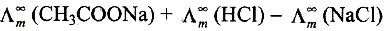= 91 + 426.16 - 126.45
= 390.71 ohm-1 cm2 mol-1

Q.2. The molar conductivity of 0.007 M acetic acid is 20 S cm2 mol-1. What is the dissociation constant of acetic acid? Choose the correct option.     (2021)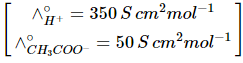(a) 1.75 × 10–5 mol L–1
(b) 2.50 × 10–5 mol L–1
(c) 1.75 × 10–4 mol L–1
(d) 2.50 × 10–4 mol L–1
Ans:
AQ.3. Hydrolysis of sucrose is given by the following reaction.      (2020)
Sucrose + H2O  ⇌ Glucose + Fructose
If the equilibrium constant (Kc) is 2 × 1013 at 300 K, the value of ΔrGº at the same temperature will be:
A: 8.314 J mol-1 K-1 × 300 K × ln(3 × 1013)
B: -8.314 J mol-1 K-1 × 300 K × ln(4 × 1013)
C: -8.314 J mol-1 K-1 × 300 K × ln(2 × 1013)

D: 8.314 J mol-1 K-1 × 300 K × ln(2 × 1013)
Ans: C
ΔG°= -RT ln K
⇒ ΔG° = -8.314 J mol-1 K-1 × 300 K-1 × ln (2 × 1013)

Q.4. On electrolysis of dil. sulphuric acid using platinum (Pt) electrode, the product obtained at the anode will be:     (2020)
A: H2S gas
B: SO2 gas
C: Hydrogen gas
D: Oxygen gas
Ans: D

Anode : 2H2O → O2 + 4H+ + 4e-
Cathode : 2H+ + 2e- → H2(g)
∴ oxygen gas will be obtained at anode.

Q.5. The number of Faradays (F) required to produce 20g of calcium from molten CaCl2 (Atomic mass of Ca = 40 g mol-1) is:     (2020)
A: 3
B: 4
C: 1
D: 2

Ans: C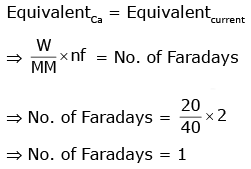Q.6. For a cell involving one electron E°cell = 0.59 V at 298 K, the equilibrium constant for the cell reaction is :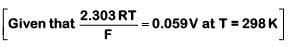(2019)
A: 1.0 × 102
B: 1.0 × 105
C: 1.0 × 1010

D: 1.0 × 1030
Ans:
C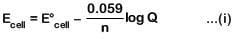(At equilibrium, Q = Keq and Ecell = 0)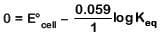(from equation (i))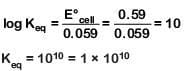Q.7. For the cell reaction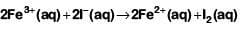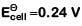at 298 K. The standard Gibbs energy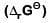of the cell reaction is :[Given that Faraday constant F = 96500 C mol–1]    (2019)
A: – 46.32 kJ mol–1
B: – 23.16 kJ mol–1
C: 46.32 kJ mol–1
D: 23.16 kJ mol–1
Ans:
A= – 2 × 96500 × 0.24 J mol–1
= – 46320 J mol–1
= – 46.32 kJ mol–1

Q.8. Consider the change in the oxidation state of Bromine corresponding to different emf values as shown in the diagram below: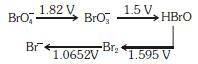Then the species undergoing disproportionation is:-    (2018)
A: BrO3-
B: BrO4-
C: Br2
D: HBrO
Ans:
D
Calculate E°cell corresponding to each compound undergoing disproportionation reaction. The reaction for which E°cell comes out +ve is spontaneous.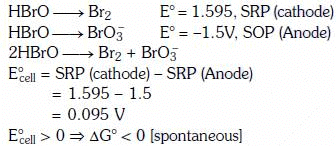Q.9.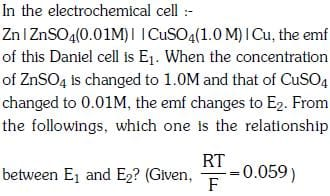(2017)
A: E1 < E2
B: E1 > E2
C: E2 = 0 ≠ E1
D: E1 = E2
Ans:
B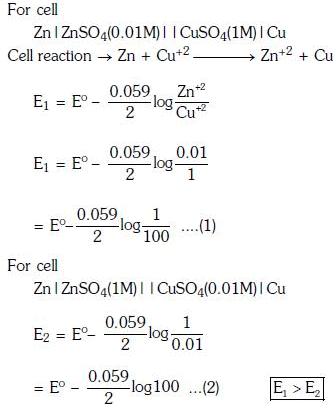Q.10. The pressure of H2 required to make the potential of H2-electrode zero in pure water at 298 K is :    (2016)
A: 10-4 atm
B: 10-14 atm
C: 10-12 atm
D: 10-10 atm
Ans:
B
pH = 7 for water.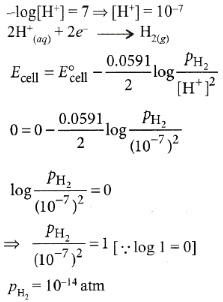Q.11. A device that converts the energy of combustion of fuels like hydrogen and methane, directly into electrical energy is known as (2015)
A: Ni-Cd cell
B: Fuel Cell
C: Electrolytic Cell
D: Dynamo
Ans:
B
In fuel cell energy of combustion is converted into electrical energy.

Q.12. When 0.1 mol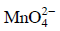is oxidised the quantity of electricity required to completely oxidiseis :    (2014)
A: 9650 C
B: 96.50 C
C: 96500 C
D: 2 × 96500 C
Ans: A

The oxidation reaction is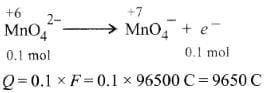Q.13. The weight of silver (at. wt. = 108) displaced by a quantity of electricity that displaces 5600 mL of O2 at STP will be :    (2014)
A: 54.0 g
B: 108.0 g
C: 5.4 g
D: 10.8 g
Ans:
B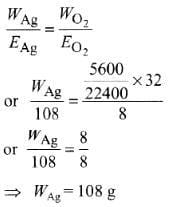The document NEET Previous Year Questions (2014-21): Electrochemistry Notes | Study Chemistry Class 12 - NEET is a part of the NEET Course Chemistry Class 12.
All you need of NEET at this link: NEETUse Code STAYHOME200 and get INR 200 additional OFF

## Chemistry Class 12

111 videos|306 docs|259 tests

### How to Prepare for NEET

Read our guide to prepare for NEET which is created by Toppers & the best Teachers

Track your progress, build streaks, highlight & save important lessons and more!

,

,

,

,

,

,

,

,

,

,

,

,

,

,

,

,

,

,

,

,

,

;